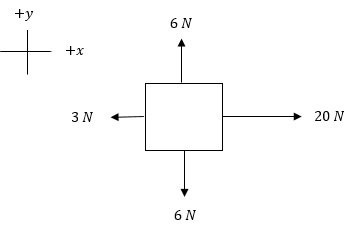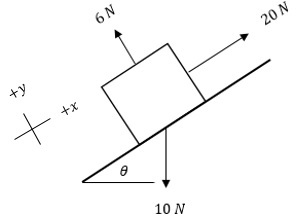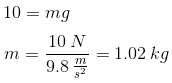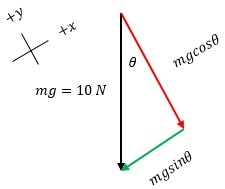# How to Use Free-Body Diagrams to Solve Motion Problems

An error occurred trying to load this video.

Try refreshing the page, or contact customer support.

Coming up next: Magnitude & Direction of the Electric Force on a Point Charge

### You're on a roll. Keep up the good work!

Replay
Your next lesson will play in 10 seconds
• 0:00 The Visual Component
• 1:44 Example 1
• 2:23 Example 2
• 4:15 Example 3
• 5:27 Lesson Summary
Save Save

Want to watch this again later?

Timeline
Autoplay
Autoplay
Speed Speed Audio mode

#### Recommended Lessons and Courses for You

Lesson Transcript
Instructor: Matthew Bergstresser

Matthew has a Master of Arts degree in Physics Education. He has taught high school chemistry and physics for 14 years.

The free-body diagram is a powerful way to analyze forces in a scenario. This lesson describes the rules you should follow when using a free-body diagram, and includes multiple examples modeling how to solve force problems.

## The Visual Component

Newton's second law of motion is one of the most famous physics equations along with Einstein's E = mc2. Even though ΣF = ma may look like a simple equation, it's actually quite sophisticated because of the sigma in front of the F. This sigma means that all forces acting on an object must be added together in each respective direction independently. Keeping track of the multitude of forces that are acting on an object is the challenge when solving problems related to force and acceleration. A free-body diagram is a way to visually inventory these forces, and helps with developing the algebraic equations needed to solve these types of problems.

There are five rules for drawing free-body diagrams:

1. To represent the object the forces are acting upon, draw either a dot or a box. It's frowned upon to depict the actual object. For example, if the object is a car, resist the temptation to draw a sketch of the car. The point of the free-body diagram is to keep any scenario, as Einstein supposedly mused, 'as simple as possible, but not simpler.'
2. Only include forces in the diagram, and represent them with arrows. No velocity vectors, acceleration vectors, or any other vectors should be drawn on the dot or box.
3. The length of the arrows should represent the relative magnitude of the force. They don't have to be to scale, though.
4. Include a coordinate system with one of the axes parallel to the direction of the acceleration.
5. All force vectors (arrows) should be pure forces, not component forces. In other words, don't use trigonometric functions in the free-body diagram.

## Example 1

Let's go through a few examples involving the use of a free-body diagram.

• Analyze the free-body diagram and determine the net force on the mass.Since force is a vector, we have to isolate each force based on the axis to which it's parallel. In the y-direction, there's a 6 N force acting in the positive direction and a 6 N force acting in the negative direction. These forces cancel, so there's no acceleration in the vertical direction. In the x-direction, there's a 20 N force acting to the right and a 3 N force acting to the left. The difference between these two forces is 17 N acting to the right; therefore, the object is accelerating to the right.

## Example 2

• A mass is on an inclined plane that's θ = 53o above the horizontal. A 20 N force is pulling the mass up the incline while a 10 N force (gravity) is pulling it down to the earth. Determine the acceleration of the mass.The 10 N force acting down is the weight of the mass due to Earth's gravity. Since the acceleration due to Earth's gravity (g) is known, we can determine the value of the mass:To keep the free-body diagram pure, we'll create a new component-force diagram analyzing the component forces acting on the mass, both in the y-direction and the x-direction.We know the angle θ and the weight; therefore, we can determine the value of the red side of the triangle, the side adjacent to θ.

To unlock this lesson you must be a Study.com Member.

### Register to view this lesson

Are you a student or a teacher?

#### See for yourself why 30 million people use Study.com

##### Become a Study.com member and start learning now.
Back
What teachers are saying about Study.com

### Earning College Credit

Did you know… We have over 200 college courses that prepare you to earn credit by exam that is accepted by over 1,500 colleges and universities. You can test out of the first two years of college and save thousands off your degree. Anyone can earn credit-by-exam regardless of age or education level.